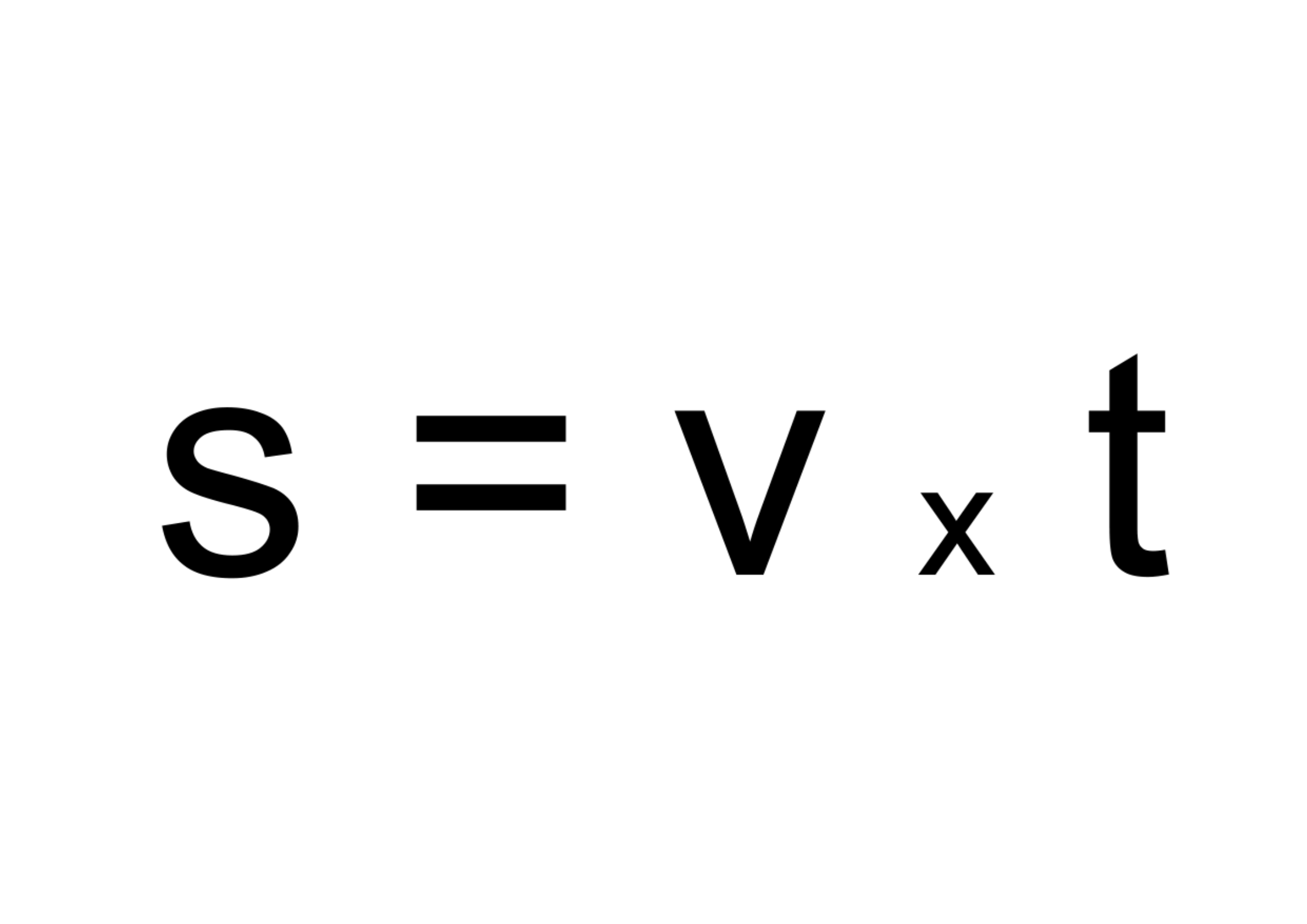﻿ Distance: - on line calculation, formula - FORMIAX

# Distances = Distance

t = Time

v = Speed

WHAT IS IT ?

Distance is the total movement of an object without any regard to direction. It is defined as how much ground an object has covered despite of its starting or ending point.

Displacement is defined as the change in position of an object. It is of vector quantity and has a direction and magnitude. It is represented as an arrow that points from the starting position to the final position.

CALCULATION:

Enter time in secons (s) od hours (h) e.g.: s

Enter velocity in metre per second (m/s) or kilometer per hour (km/h) e.g.: m/s

Enter time

Enter velocity

YOU MIGHT BE INTERESTED: